## Franklin Pezzuti Dyer

### A temporal system of logic

There's an idea that goes back to Greek philosophy that the essential nature of truth is that it is that which persists through time while everything else changes. This idea appeals to me both as a mathematician and as a computer scientist. From what I can tell, the main motivation for writing a formal proof of some logical or mathematical fact is the certainty of being able to count on that fact forever. There's the platonic philosophy that asserts the existence of a mathematical truth that has existed forever and whose veracity doesn't depend on who is aware of it, but what good to us is a fact that we can't get our hands on? Why would we assume that some assertion is even true or false at all before we know which it is?

Well, I've never been very platonically inclined but the point of this post isn't to argue about the advantages of constructivist versus platonist metaphysics. Instead, I'll describe an attempt to redesign classical propositional logic (and briefly, set theory) to make it more compatible with the constructivist viewpoint, which may even be of interest to a platonist if only because of the variety of interesting new puzzles that it gives rise to. If this post makes you want to learn more, I'd recommend the book Topos theory by Robert Goldblatt, which is very accesible and well-written (it's the book from which I first learned about these ideas) and it covers both logic and the fundamentals of category theory.

#### Temporal truth values

To represent time, we will use a set of "moments" $\mathbb P$ with a partial order $\leq$ so that $x<y$ indicates that the moment $x$ occurs (strictly) before the moment $y$. We refer to $\mathbb P$ as a frame. If $\Sigma$ is a set of sentences that can either be true or not in each instant, we will have a function $f:\mathbb P\to 2^\Sigma$ that returns the set of sentences that are true in any given moment. Our postulate that truth must persist in time can be formalized using a simple condition on the function $f$: namely, that if $x\leq y$ then $f(x)\subseteq f(y)$.

Maybe you're wondering why we would allow $\mathbb P$ to be a partial order as opposed to a total order. What does it mean to have a frame with two "moments" of time that are incomparable, that is, such that neither one occurs earlier or later? Well, imagine a team of constructive mathematicians that goes along proving things from one moment to the next and thereby expanding their body of knowledge, as represented by the set $f(t)$. But one day they realize that their theory is not strong enough to handle all of the problems that interest them, so they'll have to add another axiom to their theory. But if they're not all in agreement about what axiom to choose, they may split themselves into various groups, each of which will supplement their mathematical explorations with a different new axiom. This rupture could be represented as a bifurcation in the frame $\mathbb P$: depending on which axioms the different groups choose, it's possible that their theories might not be mutually consistent anymore. Hence, the frame serves not only to model how a theory develops through time, but also the development of several alternative theories (or "possible realities" if you will). As a computer scientist, this construction reminds me of the diagrams that one can draw to represent the different branches of a GitHub repository - perhaps this type of logic would be useful for keeping track of the features present in different branches of a programming project.

In classical logic, there are only two truth values: a sentence can be either true or false and if we're sympathetic to constructivists we might say "not true" instead of "false". But what about in an arbitrary frame? How many "different behaviors" can a sentence $\sigma$ have in a frame $\mathbb P$? In this more complicated formalization, we have to take into account not only if a sentence is true or not, but whether it is true or not in each instant, that is, the truth value of a sentence $\sigma$ will be defined by the subset of moments of $\mathbb P$ in which $\sigma$ is true. Here's a simple example of a frame: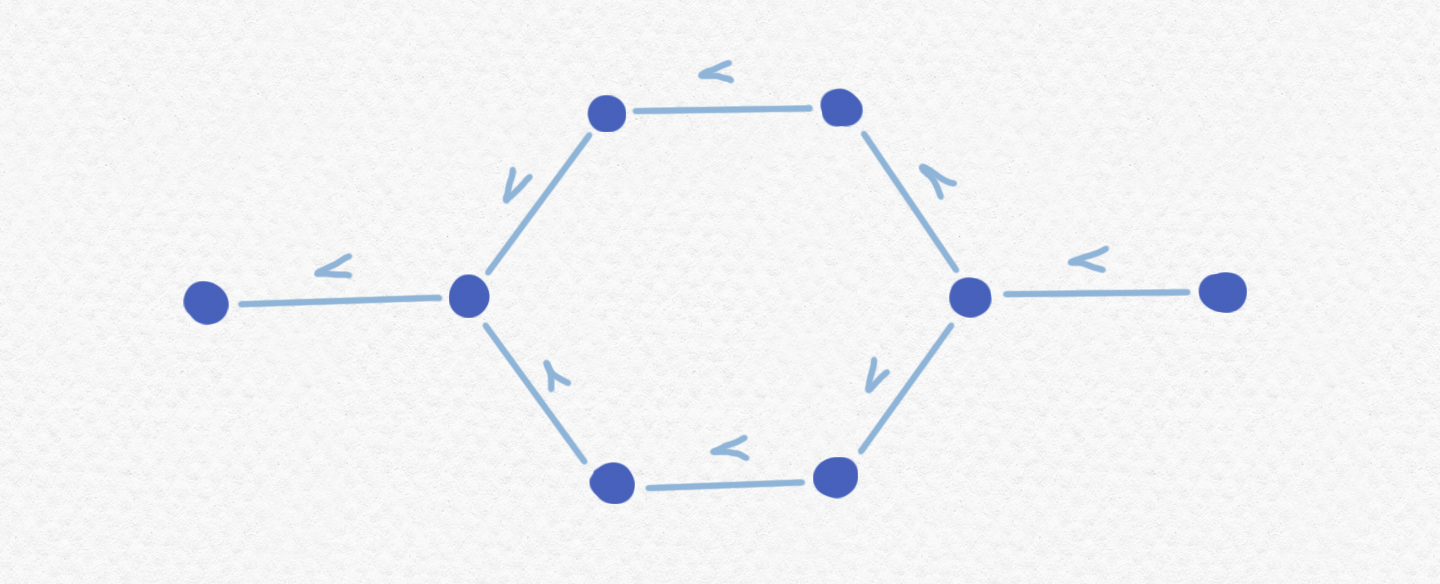Like I said, we have to consider all possible combinations of truth and non-truth in each moment of $\mathbb P$. This means that there are at most $2^{|\mathbb P|}$ truth values, but we cannot conclude that this is the exact number because some combinations will be impossible due to the requirement that truth persists over time. For instance, it's not possible for a sentence $\alpha$ to be true in the first moment but not in the last. Here's a list of all of the subsets of $\mathbb P$ that can be truth values for some sentence $\alpha$: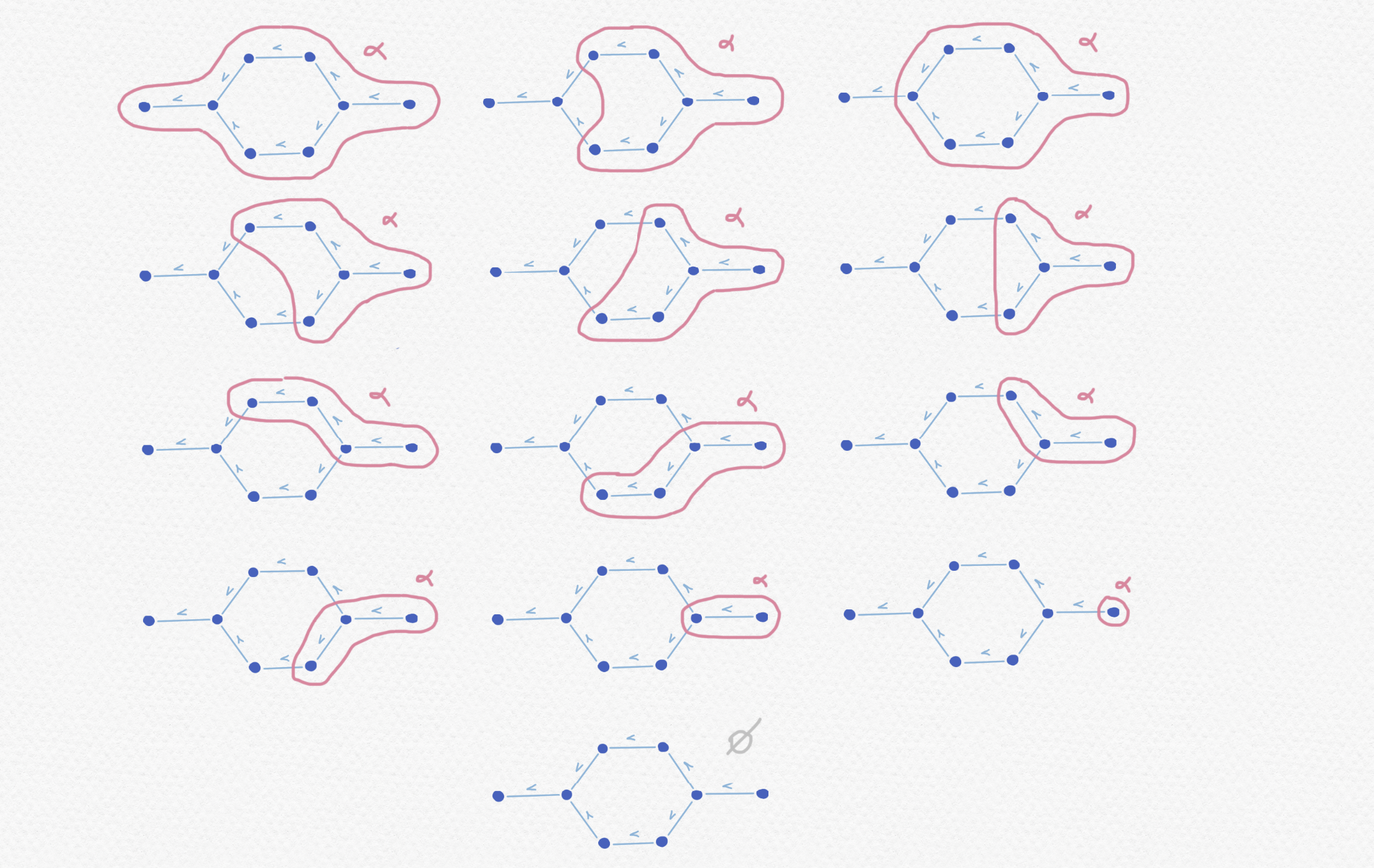In this way we can describe the truth values of a frame $\mathbb P$ as subsets $V\subset\mathbb P$ with the property that "their elements persist", that is, $x\in V$ implies that $y\in V$ for all $y\ge x$. This kind of subset of a partially ordered set is sometimes called an upper set.

Notice that even from this fairly simple frame $\mathbb P$ we get as many as $13$ different truth values. As we've already noticed, it's not possible to have more than $2^{|\mathbb P|}$ truth values, but on the other hand, for each $n$ there does exist a frame $\mathbb P$ with $n$ elements which has exactly $2^n$ truth values (namely the trivial partial order in which no two elements are comparable). Hence, the complexity of calculating the truth values of a (finite) frame can increase exponentially. In any case, there are ways of determining the truth values of a frame much more efficiently than brute force does. For instance, if I'm not mistaken, the number of different upper sets of the frame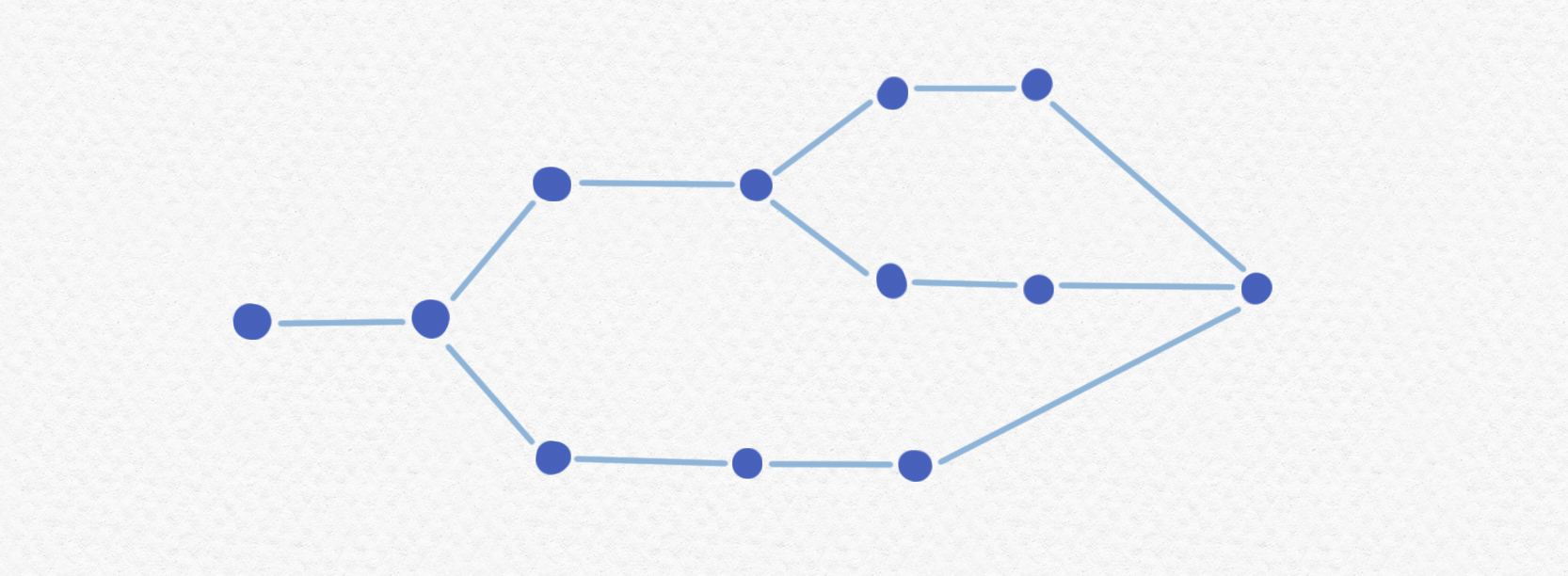will be $47$. Can you confirm this number?

#### Semantics and logical operations

If we want this system to be a viable alternative to classical logic, we'll need to define operations that correspond to the classical logical operations of "and", "or", "not" and "if-then" in order to be able to do calculations with truth values. Since these are identified with upper sets of the frame $\mathbb P$ we can define them in terms of set operations, keeping in mind that the result of applying each operation to one or two upper sets must produce another upper set (as opposed to some other subset of the frame that isn't an upper set).

If $\alpha$ and $\beta$ are sentences with truth values $U,V\subset\mathbb P$ we can define the truth values of $\alpha\land\beta$ and $\alpha\lor\beta$ easily using the operations of intersection and union of sets. We will define the truth value of $\alpha\land\beta$ as $U\cap V$ or the subset of $\mathbb P$ consisting of the moments in which both $\alpha$ and $\beta$ are true. If $\alpha$ and $\beta$ are both true in some instant then they will clearly both be true in all instants that follow since each one of them persists through time, meaning that $U\cap V$ will be an upper set as desired. Similarly, if $\alpha$ is true or $\beta$ is true in some moment then one of the two will also be true in each moment of the future, in particular the same one as whichever is true in the moment in question. Thus, we define the truth value of $\alpha\lor\beta$ as $U\cup V$ which is another upper set. Note that the upper sets form a lattice since we can take their unions and intersections.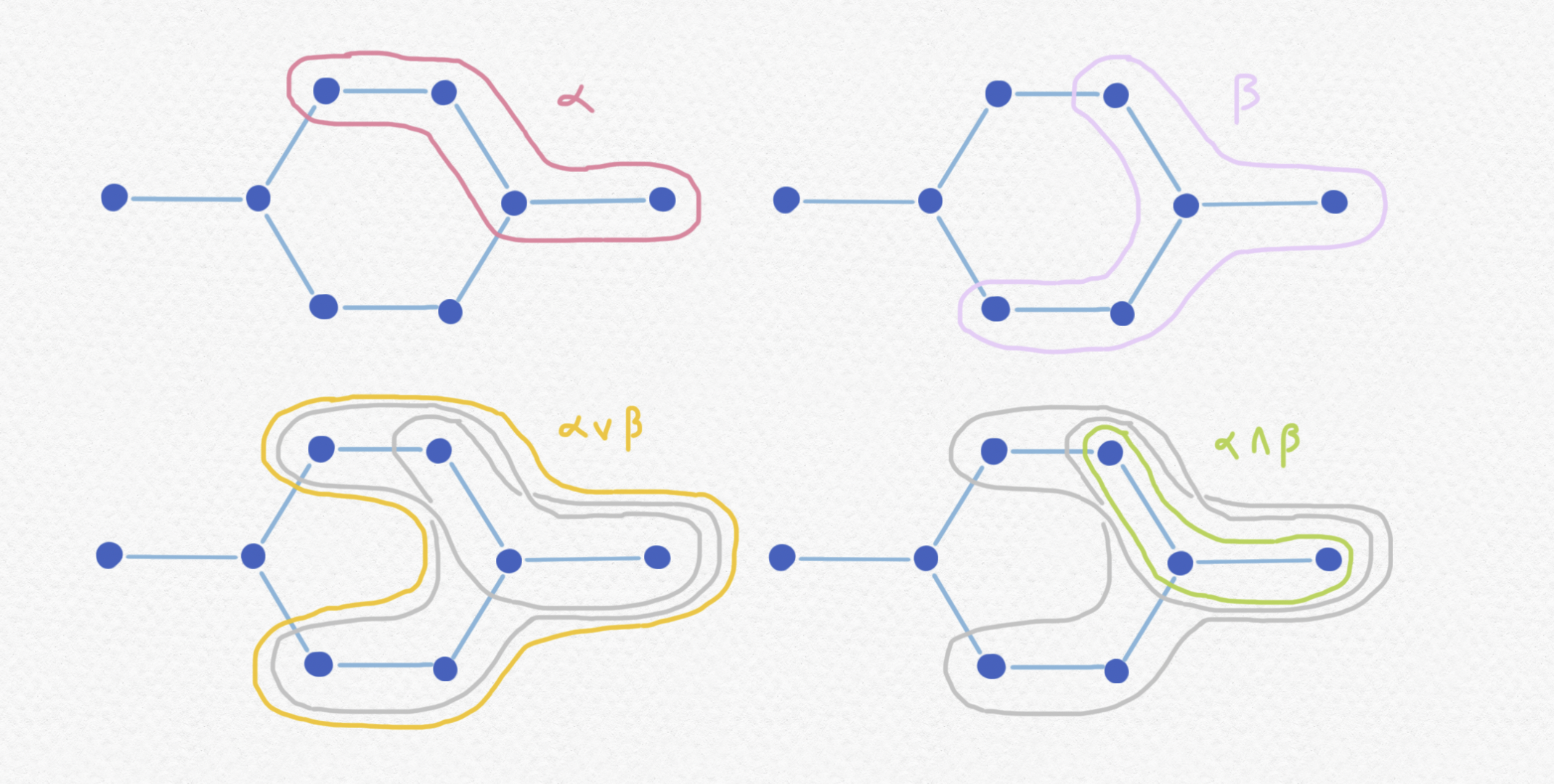Defining implication $\to$ and negation $\neg$ isn't quite as simple. The most naive attempt to define the truth value of $\neg\alpha$ would be as the set of moments in which $\alpha$ is not true, that is, the complement of the subset $U\subset\mathbb P$. But if we define it this way, there's no guarantee that this will be an upper set: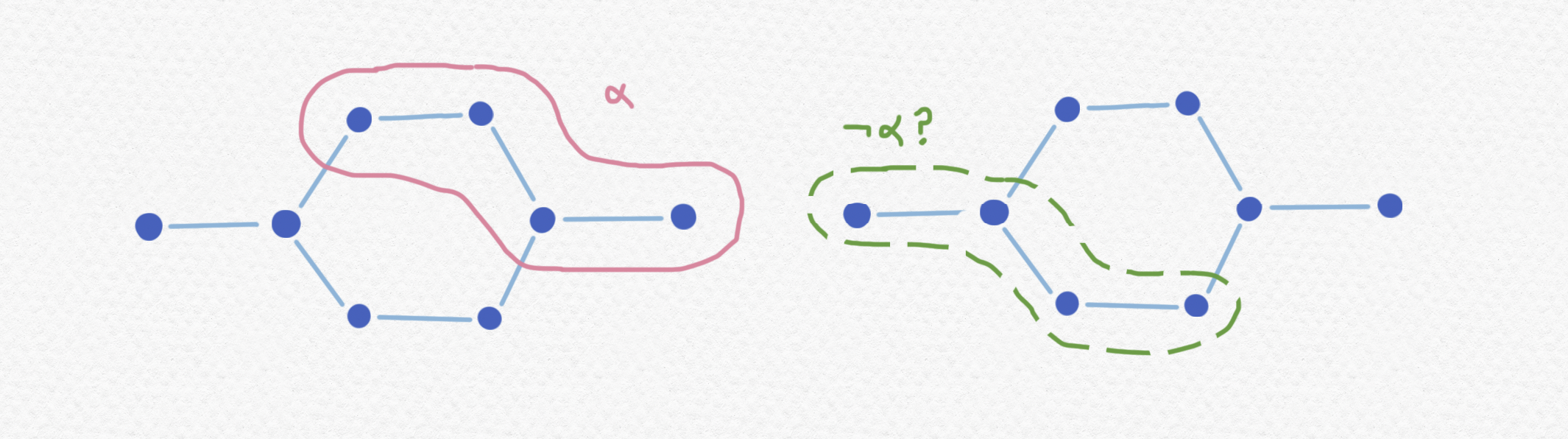In fact, we can use our postulate that truth must persist through time to put restrictions on when it's possible for the sentence $\neg\alpha$ to be true. If $\alpha$ is true in some moment $t\in\mathbb P$ then we know that $\neg\alpha$ cannot be true in any moment that shares a common future with $t$, that is, if $\neg\alpha$ is true at $u\in\mathbb P$ then there should not exist any $v\in\mathbb P$ such that $v\ge t$ and $v\ge u$. For if it existed, then it would happen that $\alpha$ and $\neg\alpha$ would both have to be true simultaneously at moment $u$ and we certainly don't want to allow a contradiction like this.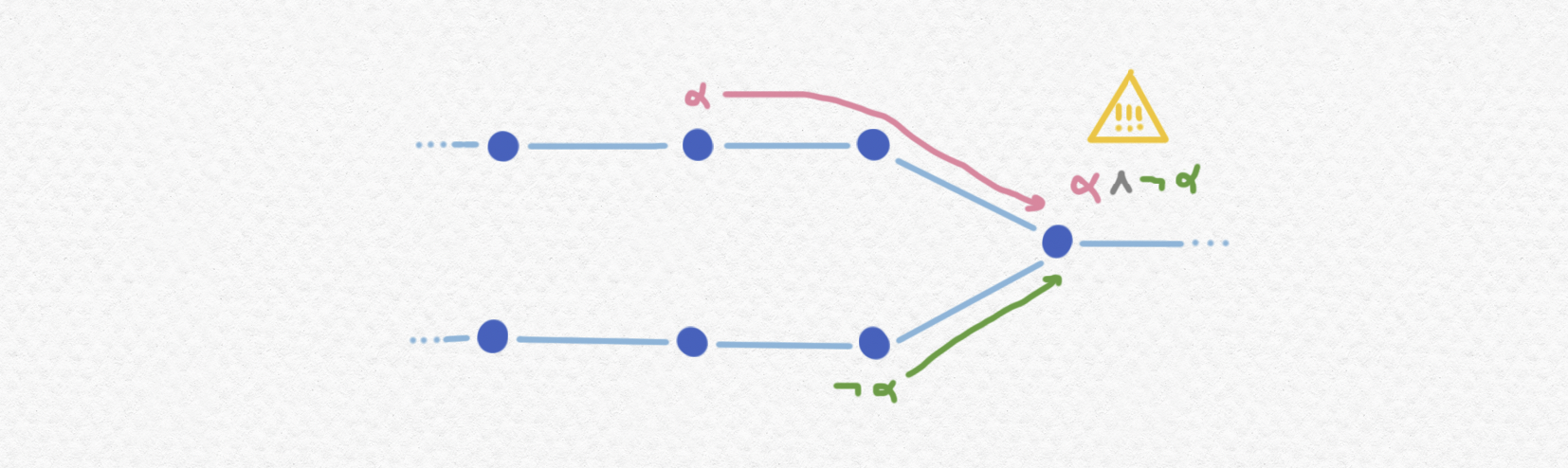Keeping this restriction in mind, why not define $\neg\alpha$ as liberally as possible without producing a contradiction, and let the truth value of $\neg\alpha$ be simply the set of elements of $\mathbb P$ that have no possible future in which $\alpha$ is true? That is, we'll define the truth value of $\neg\alpha$ like this: With this definition, the intuitive meaning of $\neg\alpha$ isn't described very aptly by "not $\alpha$" but rather perhaps something like "never $\alpha$" - a proof of $\neg\alpha$ doesn't just mean that $\alpha$ is not true in this instant, but rather that it can't become true in any possible future from this point onward either. Note that this set will indeed be an upper set because the moments that come after some moment also come after all of the moments preceding it (by the transitive property of a partial ordering) meaning that if $\alpha$ is not true in any possible future of some moment, it won't be true in the future of any of its futures either. Here's an example of how we can calculate the truth value of $\neg\alpha$ from that of $\alpha$: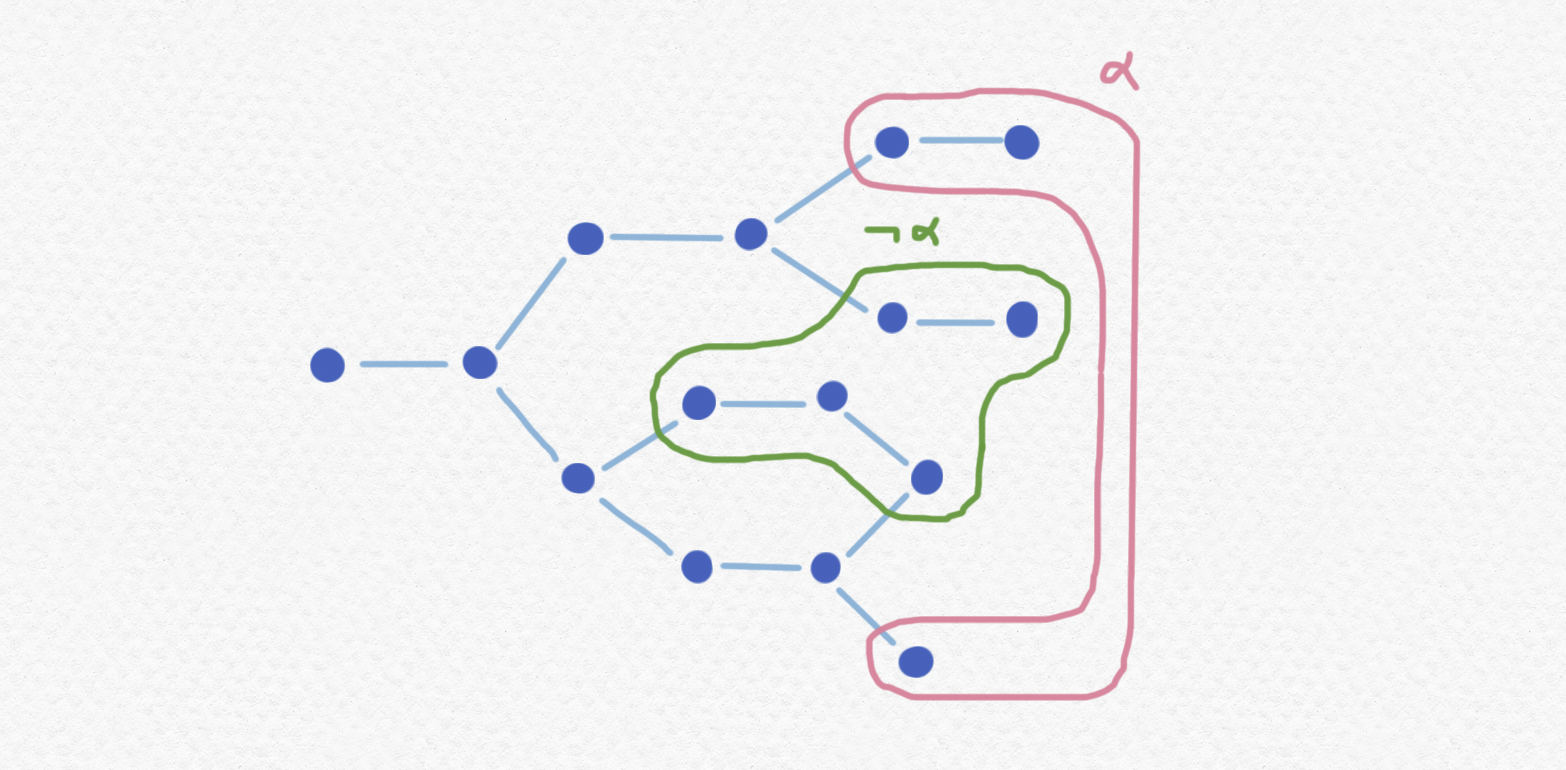We'll define the value of the implication $\alpha\to\beta$ with a similar construction. We will say that $\alpha\to\beta$ is true at the moment $t\in\mathbb P$ if $\beta$ is true in all future moments of $t$ in which $\alpha$ is true. Intuitively, it would be more apt to read $\alpha\to\beta$ as "$\beta$ as soon as $\alpha$" rather than "$\alpha$ implies $\beta$". Notice that if $\bot$ is a sentence whose truth value is equal to the empty set, then the value of $\alpha\to\bot$ will be the same as $\neg\alpha$.

#### Tautologies and Heyting algebras

In classical logic there are formulas called tautologies such as $\alpha\to\alpha$ and $\alpha\lor\neg\alpha$ that will be true regardless of the truth value of $\alpha$. We can generalize this concept to temporal logic: let's say that a formula depending on various sentences is tautological if and only if it will be true in all moments of $\mathbb P$ independently of truth values of the sentences involved. Note that which formulas are tautologies will depend on the frame with which we're working, and we will soon see that not all frames have the same tautologies.

Here's a list of classical tautologies: - $\alpha\lor\neg\alpha$ or the law of excluded middle
- $(\neg\alpha)\lor (\neg\neg\alpha)$
- $\neg(\alpha\land\neg\alpha)$ or the principle of noncontradiction
- $\neg\neg\alpha\to\alpha$ or double negation elimination
- $\alpha\to\neg\neg\alpha$ or double negation introduction
- $\neg(\alpha\land\beta)\to (\neg\alpha)\lor(\neg\beta)$
- $(\alpha\to\beta)\lor (\beta\to\alpha)$

Note that the principle of noncontradiction will continue to be a tautology in all frames, after all we've define $\neg\alpha$ with the goal of avoiding violations of this law. Double negation introduction will also work out in all frames $\mathbb P$ because $\neg\neg\alpha$ will be true in all moments at which $\alpha$ is true, for if $\alpha$ is true then it will continue to be true through all future moments meaning that $\neg\alpha$ cannot be true at any of them. But notice that the law of noncontradiction is not tautological in all frames. For instance, in the example we saw earlier: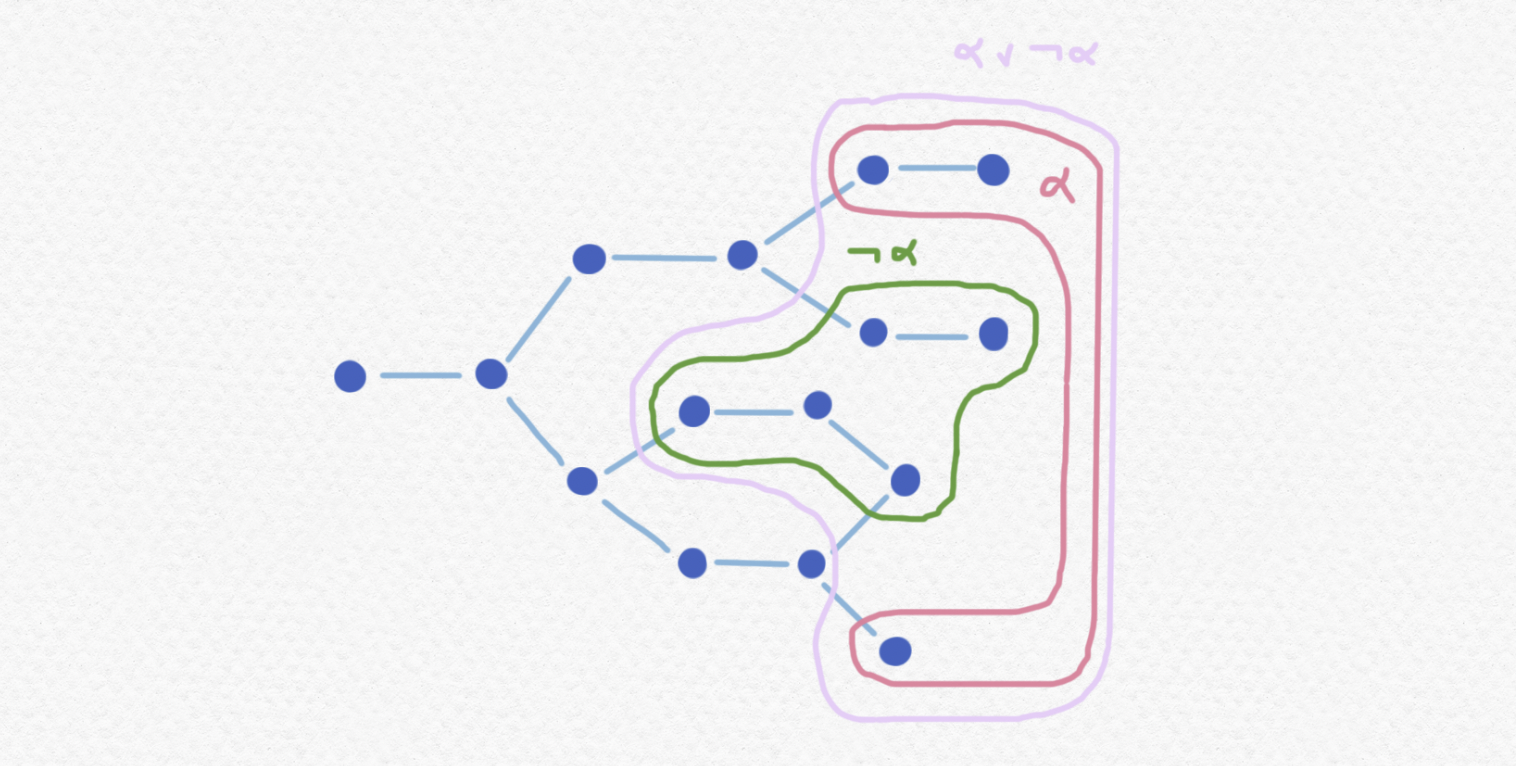We don't even need a very complicated frame $\mathbb P$. Perhaps the most simple frame that lacks the law of excluded middle will be the frame with two comparable elements. This one has $3$ truth values, the following of which does not satisfy LEM:In the first moment of $\mathbb P$, $\alpha$ is not true, but it will become true "later on" and for this reason it can't be "ruled out", so $\neg\alpha$ won't be true during the first moment either. Notice that double negation elimination won't be true in this frame either, since $\neg\neg\alpha$ is true in the first moment (since it has a possible future in which $\alpha$ is true) without $\alpha$ being true in that same moment.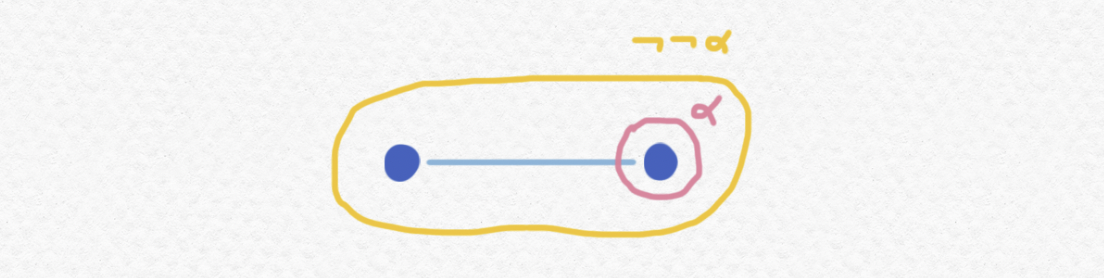In fact, we can deduce that in any frame containing $2$ comparable elements we will have a similar situation and LEM will fail, and similarly so will DNE. There are in fact several frames which don't have any pairs of distinct comparable elements, but they aren't very interesting.

Actually there is something noteworthy to say about these "degenerate" cases in which LEM and DNE are valid. If no pair of distinct elements of $\mathbb P$ is comparable, then the frame will just look like a collection of scattered points and any subset of these points will constitute an upper set, meaning that all $2^{|\mathbb P|}$ of the subsets of $\mathbb P$ will be possible truth values. The operations of $\land$, $\lor$ and $\neg$ in this case will degenerate into the usual set operations of intersection, union and complement.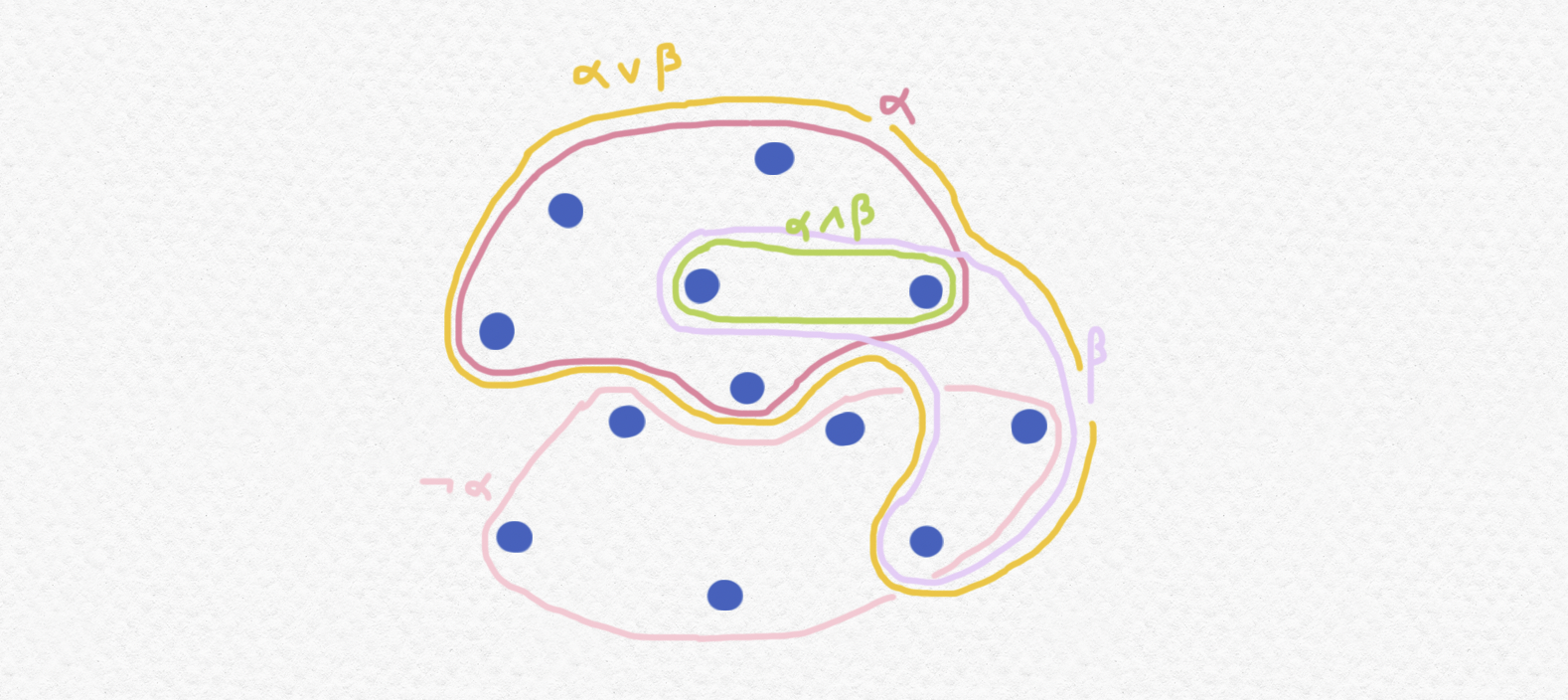If you've studied logic or set theory you might recognize this structure as a boolean algebra which is a kind of algebraic object that can be defined abstractly in terms of the identities that are satisfied by its two binary operations $\land,\lor$ and its single unary operation $\neg$ as well as the two distinguished elements $0$ and $1$. In fact, the algebraic structure formed by the truth values of an arbitrary frame (possibly nondegenerate) is less well known but also has a name: a Heyting algebra, which is defined as a lattice with operations of join/maximum $\lor$ and meet/minimum $\lor$ and maximum and minimum elements, and finally another binary operation $\to$ with the property that $(a\to b)\geq x$ if and only if $b\geq a\land x$, or equivalently such that $a\to b$ is the maximum element of the Heyting algebra $H$ such that $a\land x\leq b$. All algebras of truth values of a frame $\mathbb P$ constitute a Heyting algebra $H$, but I'll popose the following question for the reader: does every Heyting algebra arise as the algebra of truth values of some frame? Given a Heyting algebra $H$, can you find a frame $\mathbb P$ that gives rise to it? (I'd suggest that you first consider the analogous question about boolean algebras, since they're a little simpler.)

There's a lot to be said about the relationship between the nature of the partial ordering on a frame $\mathbb P$ and the tautologies of its logic. For example, we have already noticed that

$\alpha\lor\neg\alpha$ is tautological if and only if $\mathbb P$ is trivial, that is, if no two of its elements are comparable.

What structure must $\mathbb P$ have in order for $\neg\alpha\lor\neg\neg\alpha$ to be a tautology? What about $(\alpha\to\beta)\lor (\beta\to\alpha)$? If $\mathrm{LEM}(\sigma)$ denotes the sentence $\sigma\lor\neg\sigma$ and $\mathrm{DNE}(\sigma)$ denotes the sentence $\neg\neg\sigma\to\sigma$ for every sentence $\sigma$, we've already seen that there exist frames in which $\mathrm{LEM}(\alpha)$ fails to be tautological - can you find some in which $\mathrm{LEM}(\mathrm{LEM}(\alpha))$ or $\mathrm{DNE}(\mathrm{DNE}(\alpha))$ fail to be tautologies? Can you find a frame in which $\mathrm{LEM}(\alpha)$ is not a tautology but $\mathrm{LEM}(\neg\alpha)$ is a tautology?

#### Strange sets

We've already seen how logic gets more complicated if we consider different frames, but what good does this do us if we don't go on to incorporate these logics into broader theories of mathematics based upon them? It seems like the most basic thing that we'd need to construct interesting mathematical structures would be a theory of sets. How would sets behave if we were working with one of these alternative logics?

The most distinguished attempt to generalize set theory to more constructive logics is carried out using tools from category theory, and a universe consisting of objects that "behave like sets" is called a topos. The formal definition of a topos uses a lot of jargon from category theory:

A topos $\mathscr E$ is a category which has all finite limits and colimits, exponential objets and a subobject classifier.

but here's my attempt to informally describe the requirements of being a topos and their analogues in the category of sets $\mathsf{Set}$ (which is itself a topos). The existence of "limits and colimits" corresponds roughly to the existence of disjoint unions and products of arbitrary families of sets as well as quotients of sets by equivalence relations. Given two objects $X,Y$ of the category, an "exponential object" $Y^X$ plays the same role as the set of all functions between two sets in the category $\mathsf{Set}$. Finally, the concept of a "subobject classifier" is a generalization of the special relationship that exists in $\mathsf{Set}$ between the power set of a set and the set of functions from that set into the set with two elements. That is, there is a correspondence between subsets of a set and their "characteristic functions" indicating whether each element of the set is included in the subset or not. If you've seen my previous post about type theory, you could conceptualize a topos as an environment in which you can do all of the same constructions of sums, products, dependent sums and products, function types and so on.

Anyways, we don't need to understand what a topos is in general to play with different possible set theories. There's a certain family of topoi denoted $\mathsf{Set}^{\mathbb P}$ whose objects are not mere sets, but sets "that change over time" in such a way that "time" is represented again by the frame $\mathbb P$. (This is formally defined as a functor category, in case you're familiar with this construction.) The "sets" that we'll be working with if we use the frame $\mathbb P$ consist of not one single set, but rather a different set for each element of $\mathbb P$ as well as a family of functions that show how these sets are interrelated. So, to specify a set in $\mathsf{Set}^{\mathbb P}$ we have to provide the following data:

• For each $t\in\mathbb P$, a set $X_t$,
• and for each $t,u\in\mathbb P$, a function $f_{t,u}:X_t\to X_u$ such that
• for each $t,u,v\in\mathbb P$, the functions $f_{t,u}$ and $f_{u,v}$ satisfy $f_{u,v}\circ f_{t,u} = f_{t,v}$.

The final condition has to do with "coherence", that is, it requires that the functions describing "how the set changes over time" don't conflict with each other: the changes that occur between time $t$ and time $v$ are exactly the changes that occur between $t$ and $u$ combined with the changes that occur between $u$ and $v$. An "element" of such a "set" $X$ is defined as a collection of elements $x_t$ of the sets $X_t$ such that $x_t\in X_t$ for all $t\in\mathbb P$ and such that they conform to the changes described by the functions $f_{i,j}$, that is, that $f_{t,u}(x_t)=x_u$ for all $t,u\in\mathbb P$.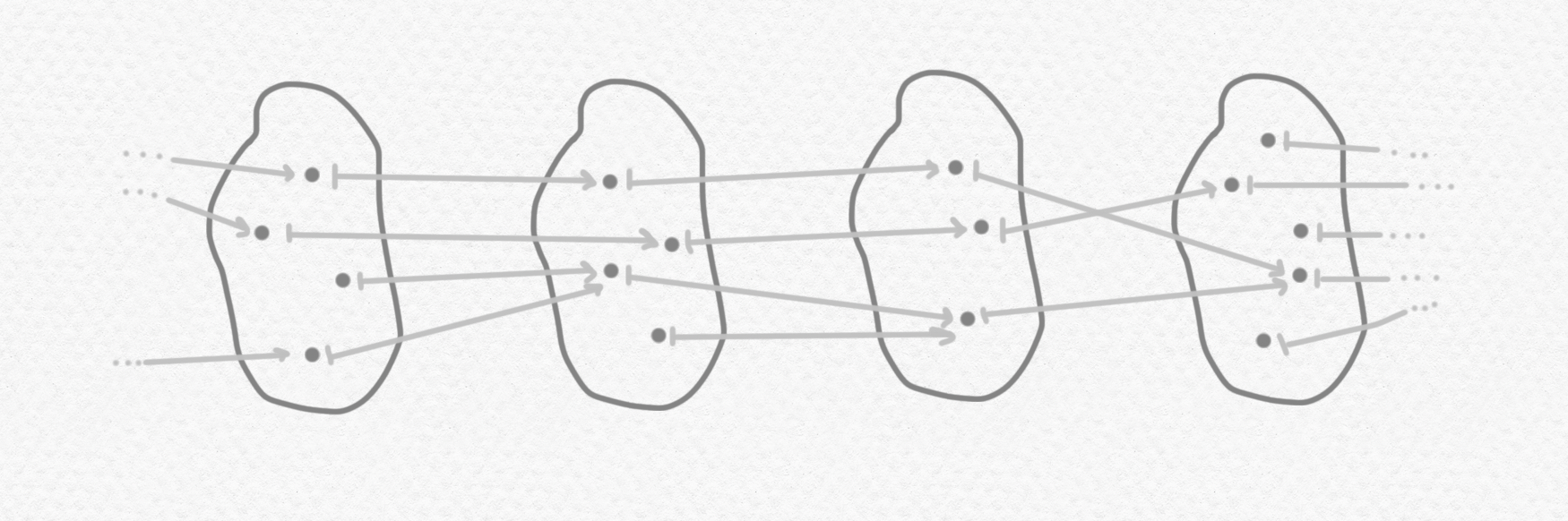Here are two elements of this "set":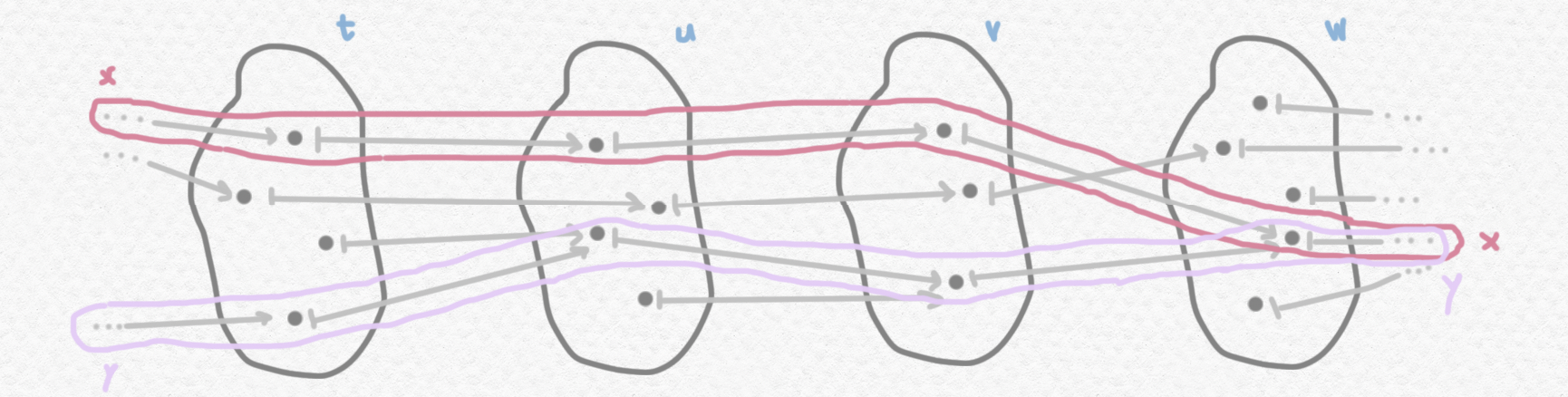The obvious way of defining equality of elements would be to declare that the sentence $(x=y)$ is true if and only if the elements of $X_t$ corresponding to $x$ and $y$ are equal at all moments $t$, that is, if $x_t=y_t$ for all $t\in\mathbb P$. Notice that if we define equality in this way, in the previous diagram the sentence $(x=y)$ is not true, but the sentence $\neg\neg(x=y)$ is true because even though $x_t=y_t$ is not true for all $t\in\mathbb P$, there does always exist a moment in the future at which they will become equal.

Even stranger things can occur with "sets through time". Consider the following example: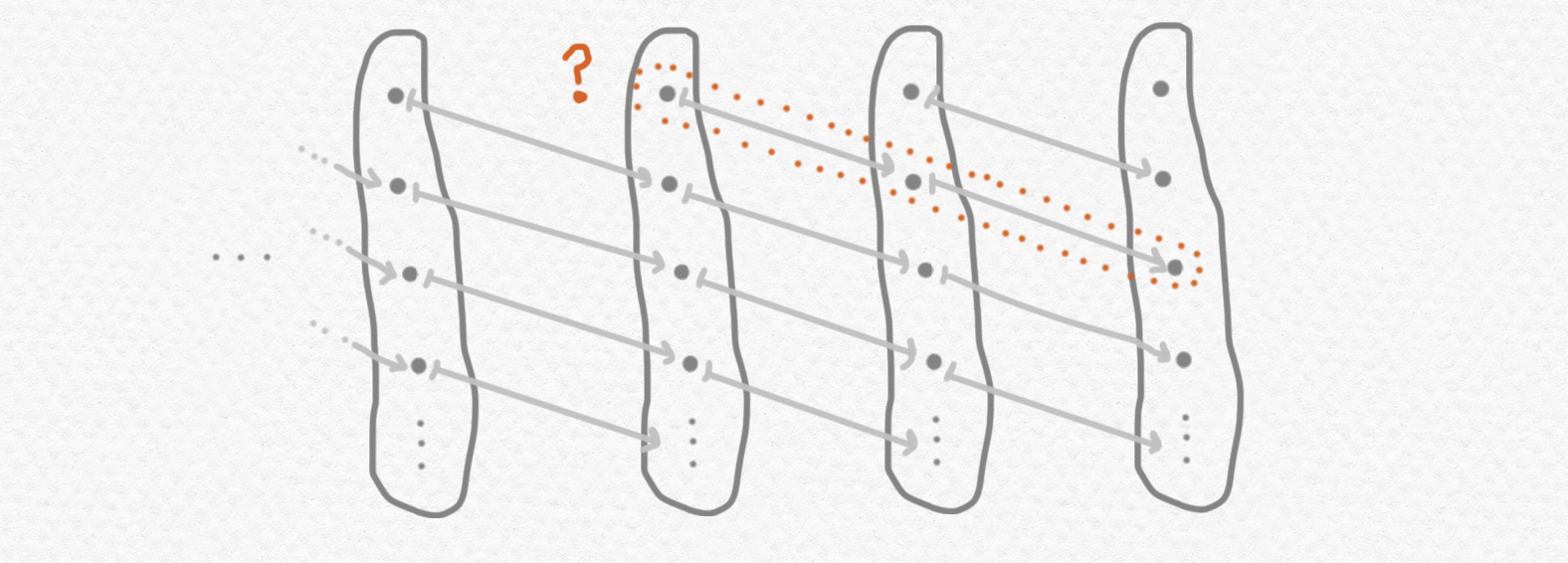Notice that this set has no elements at all. If we choose an element $x_t$ in one of the sets $X_t$ there will always exist a prior set $X_s$ with $s<t$ such that $x_t$ has no preimage in $X_s$, and hence it cannot form part of any element of $X_t$. But clearly we wouldn't want to call this set "empty"... Here's another strange example: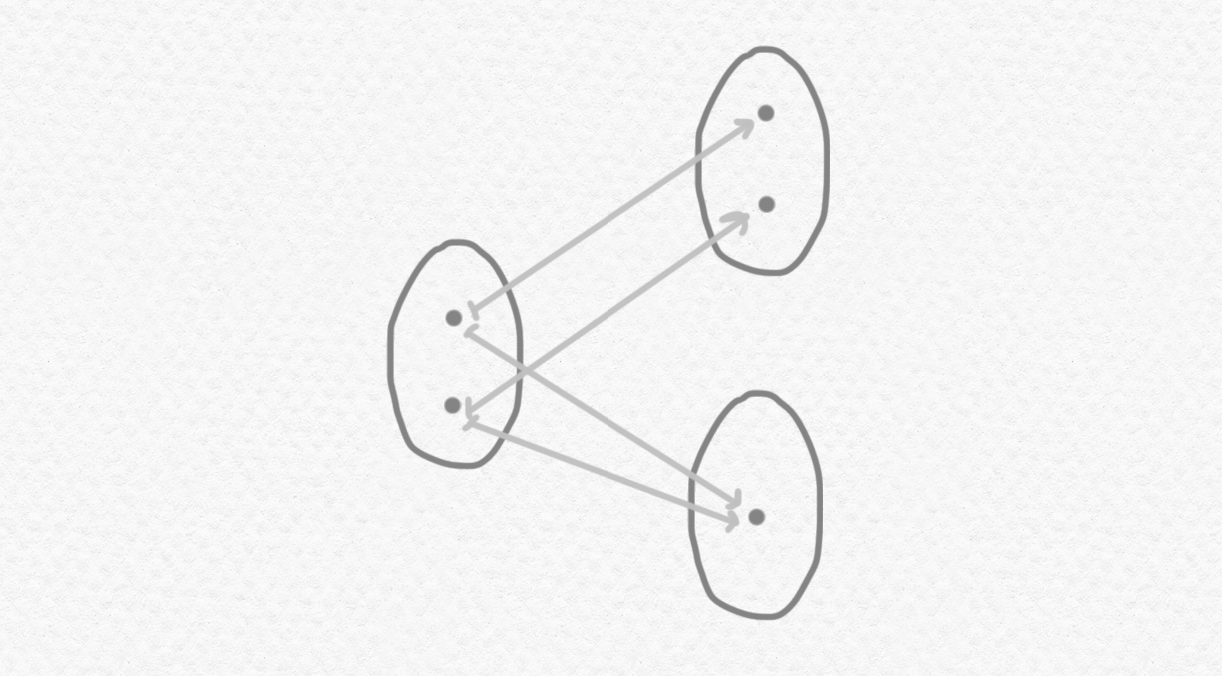This set has $2$ elements $x,y$ (can you see what they are?) but neither $(x=y)$ nor $\neg(x=y)$ nor $\neg\neg(x=y)$ nor $\mathrm{LEM}(x=y)$ is true. The blog post ends here, but if you'd like to play around a little more with these strange sets, I'd propose the following puzzles:

1. Can you find a frame $\mathbb P$ and a set $X$ of $\mathsf{Set}^{\mathbb P}$ such that each component $X_t$ is finite but the set $X$ has infinitely many elements?
2. Can you find a frame $\mathbb P$ and a set $X$ of $\mathsf{Set}^{\mathbb P}$ with three elements $x,y,z$ such that neither $\neg(x=y)$ nor $\neg(y=z)$ nor $\neg(x=y)$ is true but $\neg(x=y\land x=z)$ is true?
3. If it's possible for a set in $\mathsf{Set}^{\mathbb P}$ to have no elements even if each of its components $X_t$ is nonempty, what does this tell us about the structure of the partial ordering of $\mathbb P$?